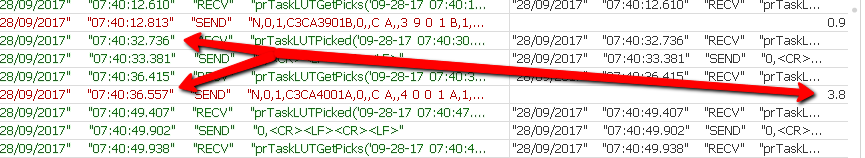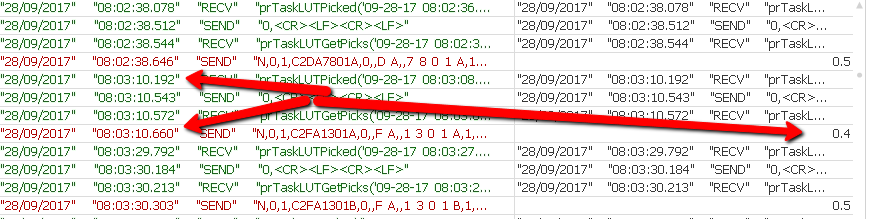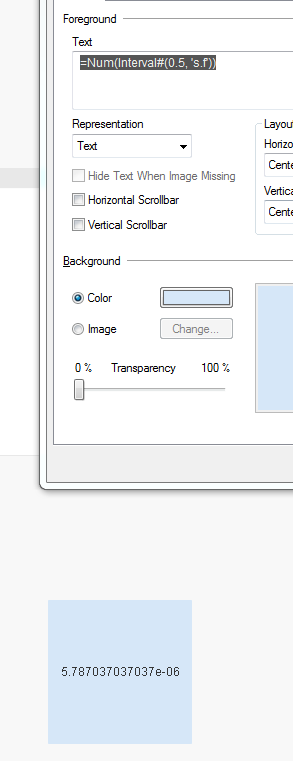# QlikView App Development

Discussion Board for collaboration related to QlikView App Development.

Honored Contributor II

## Expression Issue

Hi All,

I have the following expression.

IF(INTERVAL(\$(vTest1) - above(above(above(\$(vTest1) ))),'s.f') > \$(InitTime), INTERVAL(\$(vTest1) - above(above(above(\$(vTest1) ))),'s.f'), LogFile)

This returns a number for the time difference between transactions, so for one example it returns 3.6 (3.6 Seconds) otherwise it returns the string from the log file - which is working okThe problem I have is that it returns all times, even though my variable \$(InitTime) is set to 0.5, So I should not see 0.4, but I am seeing times less than my Variable any ideas?

Tags (4)
1 Solution

Accepted SolutionsMVP

## Re: Expression Issue

Or this

IF(INTERVAL(\$(vTest1) - above(above(above(\$(vTest1) ))),'s.f') > Interval#(\$(InitTime), 's.f'),

INTERVAL(\$(vTest1) - above(above(above(\$(vTest1) ))),'s.f'), LogFile)

12 RepliesMVP

## Re: Expression Issue

Where do you seen 0.4 in the image?

Honored Contributor II

## Re: Expression Issue

It's further down the chart, here is the image scrolled downHonored Contributor II

## Re: Expression Issue

If the time is less than 0.5 then green otherwise red for the line plus the 3 lines above

Highlighted
Honored Contributor II

## Re: Expression Issue

Hi Sunny, I know what the issue is

if I type the following expression

TIME(0.00000579,'hh:mm:ss.fff') it returns 00:00:00.500.

I am setting my variable to be .5 (500 Milliseconds), so I need to convert 0.5 back to Milliseconds

I will try that now.MVP

## Re: Expression Issue

Try this

IF(INTERVAL(\$(vTest1) - above(above(above(\$(vTest1) ))),'s.f') > Interval#(\$(InitTime)*2, 's')/2,

INTERVAL(\$(vTest1) - above(above(above(\$(vTest1) ))),'s.f'), LogFile)MVP

## Re: Expression Issue

Or this

IF(INTERVAL(\$(vTest1) - above(above(above(\$(vTest1) ))),'s.f') > Interval#(\$(InitTime), 's.f'),

INTERVAL(\$(vTest1) - above(above(above(\$(vTest1) ))),'s.f'), LogFile)

Honored Contributor II

## Re: Expression Issue

No that does not return the correct result.

I need to convert 0.5 to seconds, as Qlikview treats this as 12:00:00.000,

If I set my variable to 0.00000579, it works perfectly, but I want to convert 0.5's as a numberMVP

## Re: Expression Issue

That is what it should be doing... this give me

=Num(Interval#(0.5, 's.f'))

thisReplace 0.5 with your variable and it should work (I think)

Honored Contributor II

## Re: Expression Issue

Sorry it's working thanks